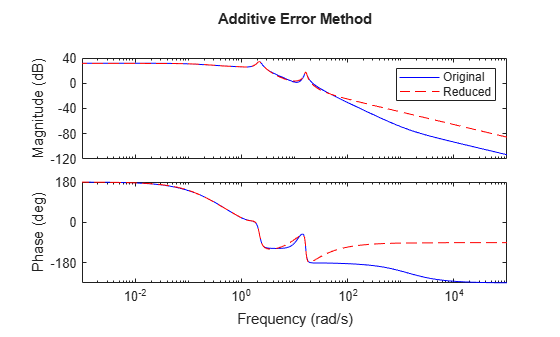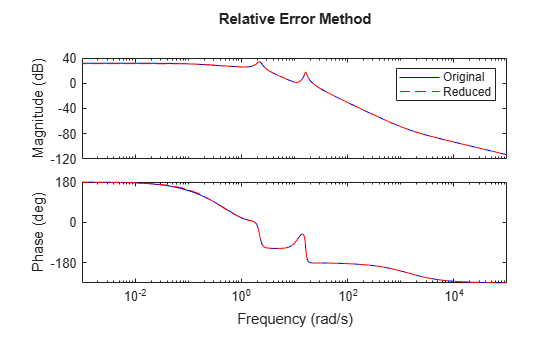# Approximate Plant Model by Multiplicative Error Method

In most cases, the multiplicative error model reduction method `bstmr` tends to bound the relative error between the original and reduced-order models across the frequency range of interest, hence producing a more accurate reduced-order model than the additive error methods. This characteristic is obvious in system models with low damped poles.

The following commands illustrate the significance of a multiplicative error model reduction method as compared to any additive error type. Clearly, the phase-matching algorithm using `bstmr` provides a better fit in the Bode plot.

```rng(123456); G = rss(30,1,1); % random 30-state model [gr,infor] = reduce(G,'Algorithm','balance','order',7); [gs,infos] = reduce(G,'Algorithm','bst','order',7); figure(1) bode(G,'b-',gr,'r--') title('Additive Error Method') legend('Original','Reduced')``````figure(2) bode(G,'b-',gs,'r--') title('Relative Error Method') legend('Original','Reduced')```Therefore, for some systems with low damped poles or zeros, the balanced stochastic method (`bstmr`) produces a better reduced-order model fit in those frequency ranges to make multiplicative error small. Whereas additive error methods such as `balancmr`, `schurmr`, or `hankelmr` only care about minimizing the overall "absolute" peak error, they can produce a reduced-order model missing those low damped poles/zeros frequency regions.